# McGraw Hill Math Grade 4 Chapter 6 Lesson 1 Answer Key Dividing a Two-Digit Number

Practice the questions of McGraw Hill Math Grade 4 Answer Key PDF Chapter 6 Lesson 1 Dividing a Two-Digit Number to secure good marks & knowledge in the exams.

## McGraw-Hill Math Grade 4 Answer Key Chapter 6 Lesson 1 Dividing a Two-Digit Number

Divide

Question 1.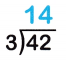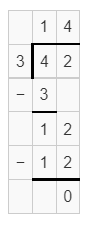Explanation:
quotient = 14
remainder = 0

Question 2.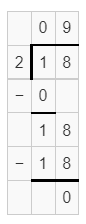Explanation:
quotient = 9
remainder = 0

Question 3.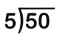Explanation:
quotient = 10
remainder = 0

Question 4.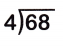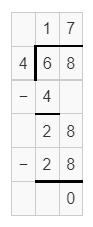Explanation:
quotient = 17
remainder = 0

Question 5.
Anwar has 42 books. He places 14 books in each box. Draw a picture to determine how many boxes Anwar used.